# Development of Self-characterization Digital Signal Processing Terminal

Author: pandoramc

Creation date:

Evaluation Type: Development Boards & Tools

Did you receive all parts the manufacturer stated would be included in the package?: True

What other parts do you consider comparable to this product?: Raspberry Pi; Xilinx Kria platform, Beagle Bone Series

What were the biggest problems encountered?: coprocessor acquisition time

Detailed Review:

## Introduction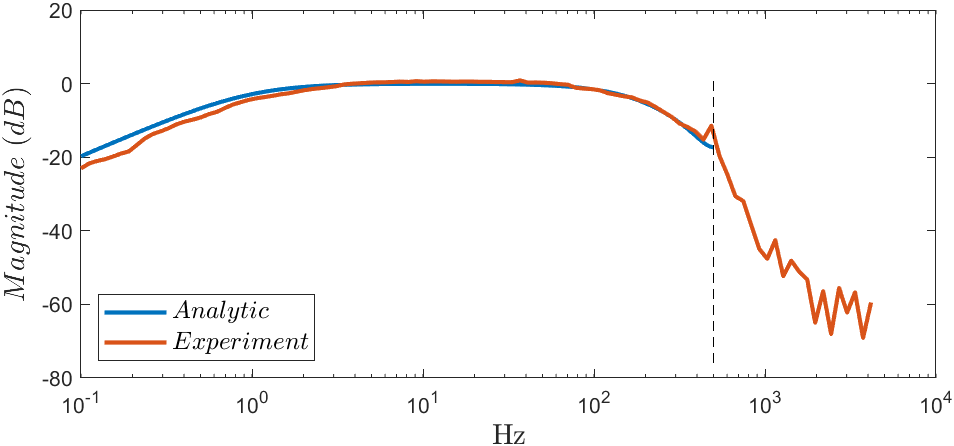The figure above represents a comparative between an ideal curve model and the experimental curve for a digital signal processor with signal conditioning. Since the discrete model behavior must be approximate to the analytical model, the microcontroller signal path to transfer equation, the output is equal to the intput, should represent the same curve. According to the figure, if the frequency overcomes a threshold, black line, the discrete system does not guarantee the behavior. Hence there rise up some questions:

## Which are the circuits involved in the signal processing path?

The first stage is the development of some signal conditioner for the input and output. The input must be posititive all the time, consequently, it is required add a reference for a pure AC signal. In the other hand, we must avoid the aliasing effect. Despite the ATmega32u4's ADC is not able to handle high frequencies, yet users connect arbitrary bandwidth signals in the digital systems. So, the following circuit is implemented to solve these problems.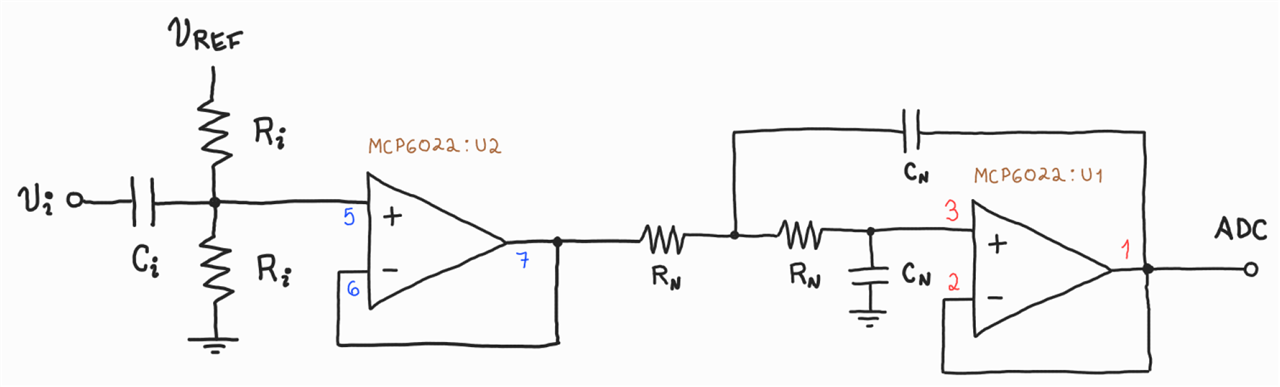Other Problem to solve is the fact that the ATmega32u4 has not a DAC. Using Fourier Series theory is possible to implement other low-pass filter to minimize the influence of the high-frequency components. This idea brings the circuit below to implement a PWM-based DAC.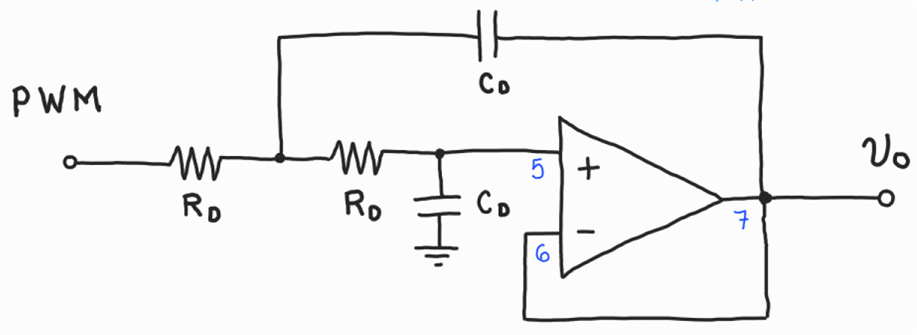In order to minimize the latency inherent to Arduino HAL, the code was implemented in hybrid way. Using bare-metal programming, the Timer0 is configured as periodic generator for ADC convertion and the Timer1 is configured as 8-bit PWM generator to create the signal path transfer and proced to characterize the DSP system.

```void setup(){
cli();
DDRB = 0B10100000;

DIDR0 = 255;

TCCR1A = 0B10000001;
TCCR1B = 0B00001001;

OCR0A = 249;
TCCR0A = 0B00000000;
TCCR0B = 0B00000011;
TIMSK0 = 0x02;

sei();
}

void loop(){}

}

ISR(TIMER0_COMPA_vect){}```

## How can I get the reference curve and experimental curve?

Each circuit has a transfer function associated. the first one consist on the high pass filter in two scenarios, when the input is AC and when is DC. The second one has a 2nd order low-pass filter, which cut-off frequency is allocated according to Nyquist theorem, to avoid the aliasing effect. Finally, other 2nd order filter is implemented to recover the DC component from the PWM signal. These facts allow implement the following transfer function.This equation creates an analytical reference for the experiment.

Note: It is important have in mind that the equation apply for signal transfer.

## Is the Latte Panda able to handle additional instruments?

Starting the Latte Panda it is possible observe that Windows OS is available. This brings the possibility install two important tools: MATLAB and Waveforms. MATLAB allows simulate the transfer function and compare the results with the experiment acquired data. The data acquisition is made with the Analog Discovery 2 and Waveforms software. These allow the following setup.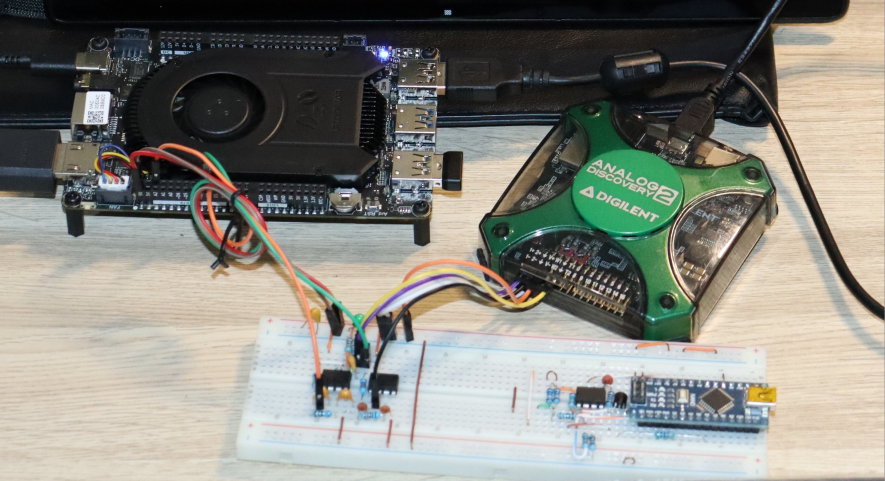The Analog Discovery has a tool called Network Analyzer. This tool is able to generate fundamental signal to test the system response to frequency. After some minutes, the system response obtained is the following,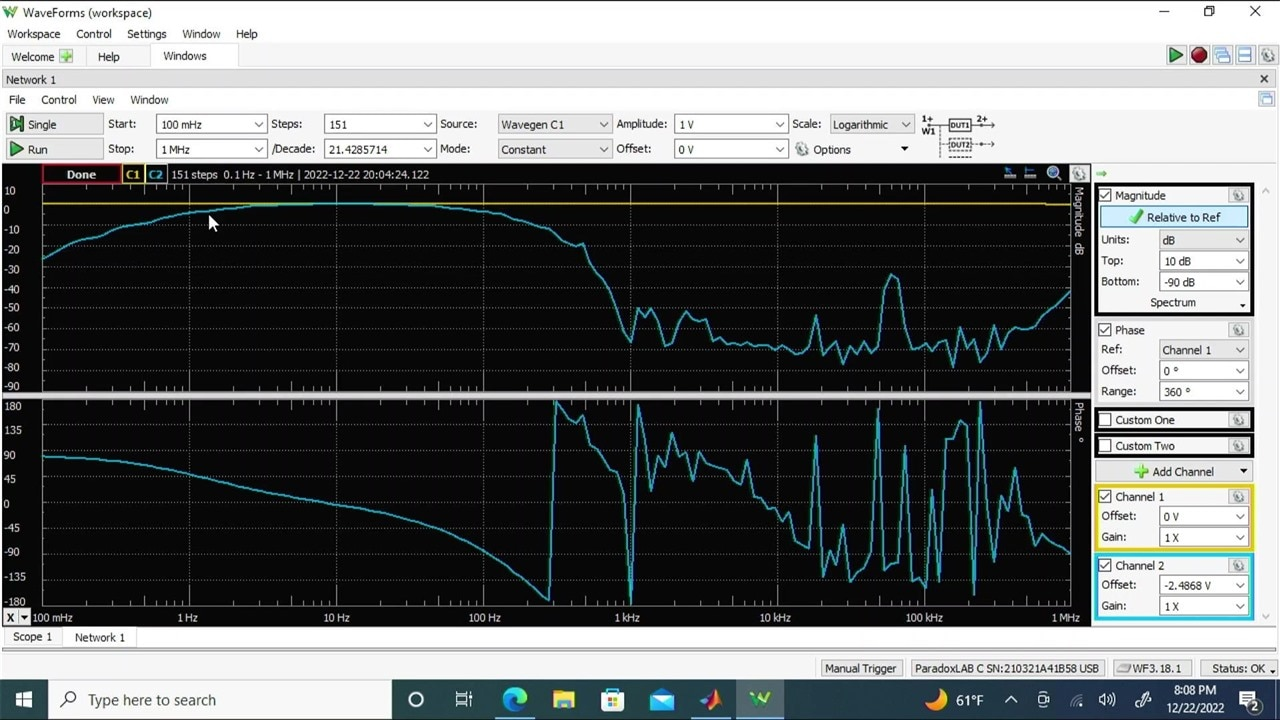MATLAB has no problems to run scripts for compare the data. Waveforms export the response data in CSV file, consequently, this information can be loaded in MATLAB interface to compare with the analytical data. These proccess achieve the response below.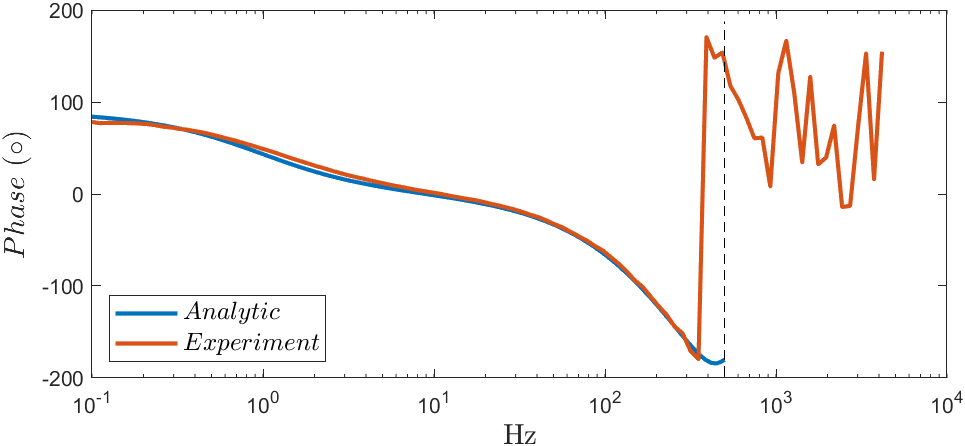## Conclusion

The system response observed, compared with the analytical response, allows correct signal processing processing from 100 mHz to 500 Hz approximately. Unfortunately, the processing time increases according the number of variables and memory requirements. Despite this limitation, the platform is able to conform a full academic system to start learning in digital signal processing. The platform together the Analog Discovery, prototyping boards and circuits, conform a powerfull tool in the school.